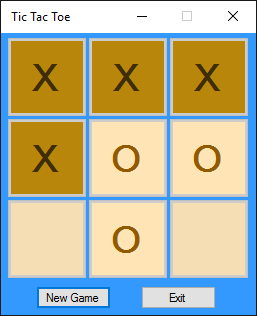Tic Tac Toe Game using C#Posted by Samath4361 January 01, 2016

This is a simple two player tic tac toe game written using C#. When the game start, player 1 start by clicking on a empty box. Each player takes turn until someone win or the game draw. Player 1 is automatically assign X and player 2 is assign O.  As i said before this is a simple game, the game has two button. New game button which resets the game and Exit button which quits the game. Feel free to extend the game in any way you want. Good Luck!Code:

```using System;
using System.Collections.Generic;
using System.ComponentModel;
using System.Data;
using System.Drawing;
using System.Linq;
using System.Text;
using System.Windows.Forms;

namespace TicTacToe
{
public partial class Form1 : Form
{
bool isTurn = true;
int Turn_Count = 0;

public Form1()
{
InitializeComponent();
}

private void button_click(object sender, EventArgs e)
{
Button b = (Button)sender;
if (isTurn)
{
b.Text = "X";
b.BackColor = Color.DarkGoldenrod;
}
else
{
b.Text = "O";
b.BackColor = Color.Moccasin;
}
isTurn = !isTurn;
b.Enabled = false;
Turn_Count++;

Winner();
}

private void Winner()
{
bool isWinner = false;

if ((A1.Text == A2.Text) && (A2.Text == A3.Text) && (!A1.Enabled))
isWinner = true;
else if ((B1.Text == B2.Text) && (B2.Text == B3.Text) && (!B1.Enabled))
isWinner = true;
else if ((C1.Text == C2.Text) && (C2.Text == C3.Text) && (!C1.Enabled))
isWinner = true;

else if ((A1.Text == B1.Text) && (B1.Text == C1.Text) && (!A1.Enabled))
isWinner = true;
else if ((A2.Text == B2.Text) && (B2.Text == C2.Text) && (!A2.Enabled))
isWinner = true;
else if ((A3.Text == B3.Text) && (B3.Text == C3.Text) && (!A3.Enabled))
isWinner = true;

else if ((A1.Text == B2.Text) && (B2.Text == C3.Text) && (!A1.Enabled))
isWinner = true;
else if ((A3.Text == B2.Text) && (B2.Text == C1.Text) && (!C2.Enabled))
isWinner = true;

if (isWinner)
{
Disable_Button();

string winner = "";
if (isTurn)
winner = "O";
else
winner = "X";

MessageBox.Show(winner + " Wins!", "Tic Tac Toe");
}
else
{
if (Turn_Count == 9)
MessageBox.Show("Draw!", "Tic Tac Toe");
}
}
private void Disable_Button()
{

foreach (Control c in Controls)
{
Button b = (Button)c;
if (b.Text != "New Game" && b.Text != "Exit")
{

b.Enabled = false;
}
}

}

private void btnExit_Click(object sender, EventArgs e)
{
Application.Exit();
}

private void btnnewGame_Click(object sender, EventArgs e)
{
isTurn = true;
Turn_Count = 0;

foreach (Control c in Controls)
{

Button b = (Button)c;
if(b.Text!= "New Game" && b.Text != "Exit")
{
b.Enabled = true;
b.Text = "";
b.BackColor = System.Drawing.Color.Wheat;
b.Font = new System.Drawing.Font("Microsoft Sans Serif", 27.75F, System.Drawing.FontStyle.Bold, System.Drawing.GraphicsUnit.Point, ((byte)(0)));
b.ForeColor = System.Drawing.Color.Cyan;
b.UseVisualStyleBackColor = false;
}

}

}

}
}
```